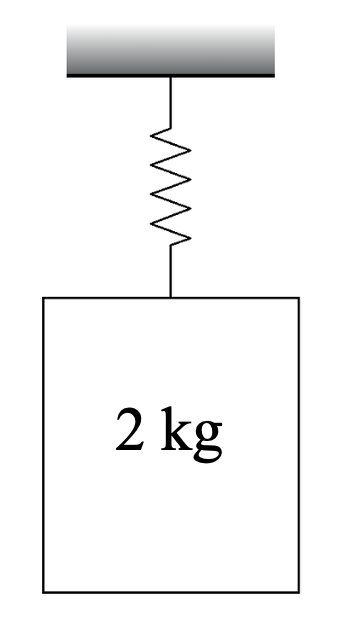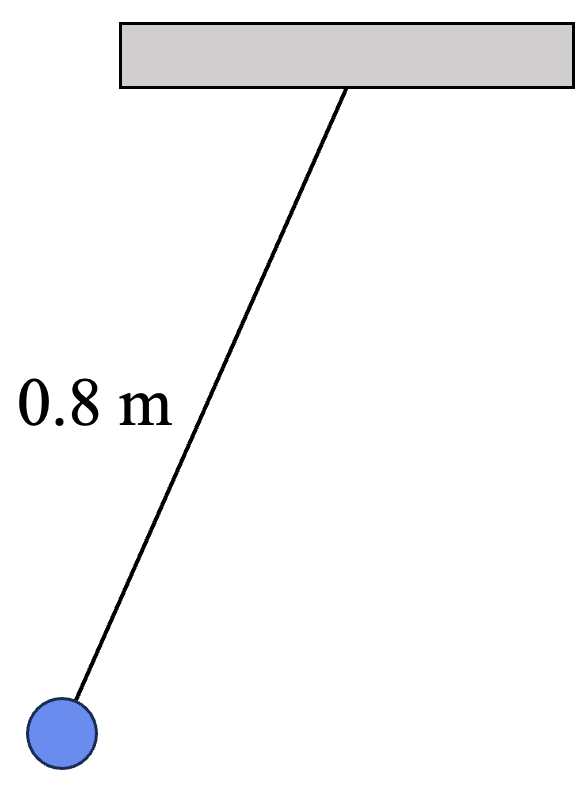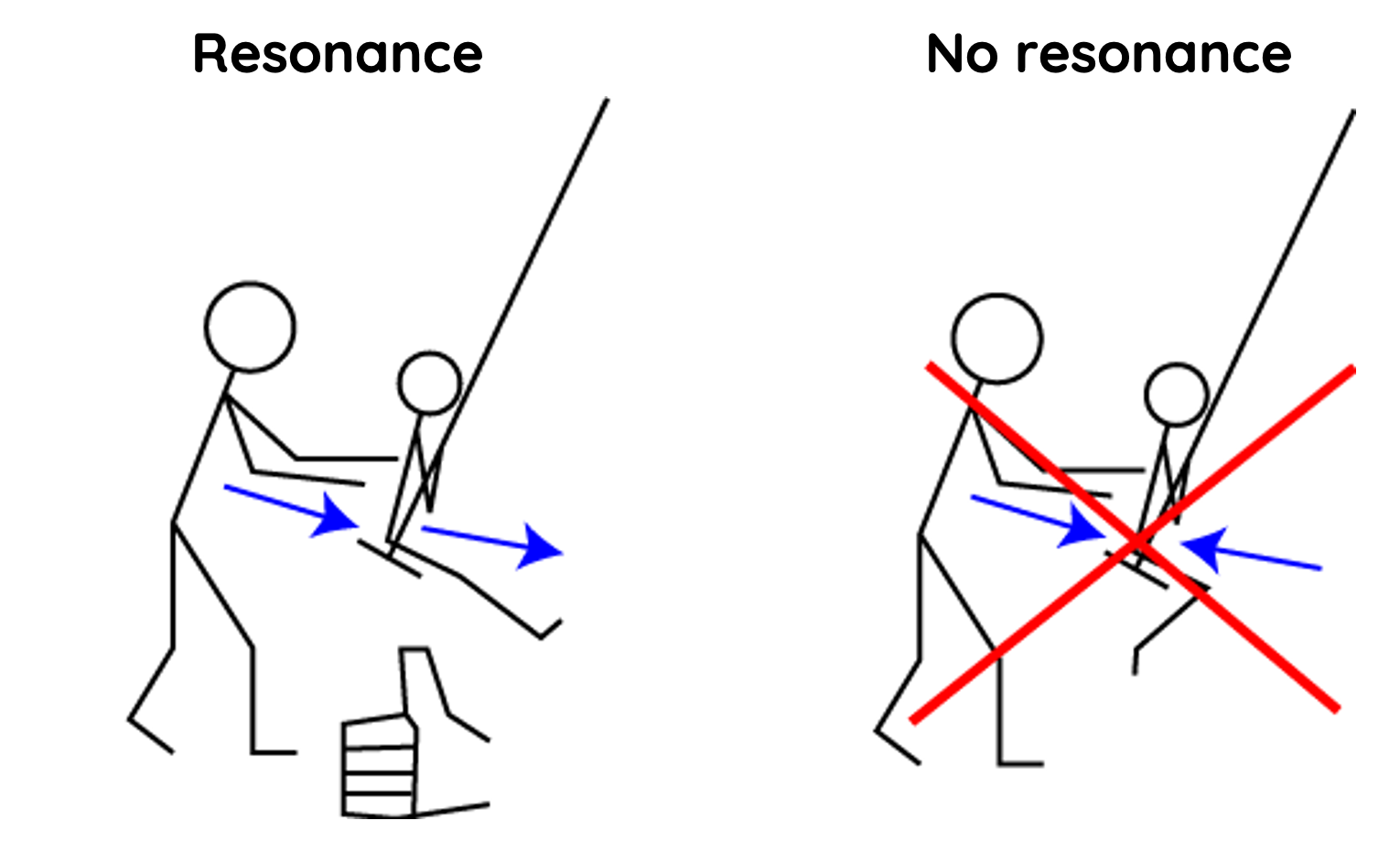# Resonance in Mechanical Systems

This topic is part of the HSC Physics course under the section Wave Behaviour.

### HSC Physics Syllabus

• conduct an investigation to explore resonance in mechanical systems and the relationships between:
– driving frequency
– natural frequency of the oscillating system
– amplitude of motion
– transfer/transformation of energy within the system (ACSPH073)

### What is Natural Frequency?

Every mechanical system, whether a swing or a guitar string, has a natural frequency at which it prefers to oscillate. Amplitude refers to the maximum displacement from a system's equilibrium position. The equilibrium position is the position at which the system is at rest.

We will examine the concept of natural frequency using a mass-spring system and a simple pendulum system below.

### Mass-spring System

The natural frequency f of a simple mass-spring system is given by:

$$f = \frac{1}{2\pi} \sqrt{\frac{k}{m}}$$

Where:

• k is the spring constant (in Newtons per metre or N/m)
• m is the mass attached to the spring (in kilograms)

The period of the mass-spring system is defined as the time taken for the mass to complete one oscillation or to return to its initial position. The period is the inverse of its frequency:

$$T = 2\pi \sqrt{\frac{m}{k}}$$

In a mass-spring system, the period and natural frequency depend on the mass and spring-constant, and are independent of the amplitude of oscillation.

In the above demonstration, the following observations are made:

• regardless of how far away from the equilibrium position the mass oscillates from, the time taken to complete one oscillation is the same.
• when a heavier mass is used, the period increases, and the natural frequency decreases.
• when two springs are used instead of one, the period decreases, and the natural frequency increases due to a greater spring constant.

#### Calculation Example 1

A 2 kg is attached to the ceiling via a spring with a spring constant k = 15 N/m.What is the natural frequency of this mass-spring system?

Solution:

$$f = \frac{1}{2\pi} \sqrt{\frac{k}{m}}$$

$$f = \frac{1}{2\pi} \sqrt{\frac{15}{2}}$$

$$f = 0.44 \, \text{Hz}$$

### Simple Pendulum Systems

With a pendulum, at its peak height (maximum amplitude), it holds maximum potential energy. As it swings down and speeds up, this potential energy transforms into kinetic energy. This energy transformation is most efficient during resonance, i.e. when the pendulum swings at its natural frequency.

The natural frequency f of a simple pendulum is related to its period T. The period T of a simple pendulum is given by:

$$T = 2\pi \sqrt{\frac{l}{g}}$$

The natural frequency f is the inverse of the period T:

$$f = \frac{1}{T} = \frac{1}{2\pi} \sqrt{\frac{g}{l}}$$

Where:

• l is the length of the pendulum (from the pivot point to the centre of mass of the pendulum bob) in meters.
• g is the acceleration due to gravity ( near the Earth's surface).

It's important to note that the above formula for the pendulum's period (and thus its frequency) assumes small angle approximations (typically less than 15 degrees).

For HSC Physics students, these equations provide a quantitative way to understand and predict the behaviour of simple harmonic oscillators.

In a simple pendulum system, the period of oscillation and natural frequency are dependent on the length of the string and gravitational acceleration; the two are independent of mass.

#### Calculation Example 2

A simple pendulum system is attached to a 0.8 m long massless string.What is the natural frequency of the oscillation on the surface of Earth?

Solution:

$$f = \frac{1}{2\pi} \sqrt{\frac{g}{l}}$$

$$f = \frac{1}{2\pi} \sqrt{\frac{9.8}{0.8}}$$

$$f = 0.56 \, \text{Hz}$$

### Driving Frequency and Resonance

Resonance occurs when an external force, termed the driving frequency, matches the natural frequency of a mechanical system.

When experiencing resonance, a system's amplitude reaches its peak because it's absorbing energy from the external force with utmost efficiency. In other words, when the matching force's driving frequency matches the system's natural frequency, the amplitude of oscillation increases.

During resonance, energy from the external force is efficiently transferred to the system. This energy alternates between potential energy (when the system is momentarily still) and kinetic energy (when in motion).

#### SwingsA child on a swing can be modelled as a simple pendulum system. Pushing the child at just the right interval (the swing's natural frequency) causes the swing to go higher and higher (increased amplitude). Push too quickly or too slowly, and the swing won't go as high (less energy is transferred).

#### Sound Wave & Glass

Consider a wine glass on a table. Every object, including the glass, has a natural frequency at which it vibrates. When a singer hits a note that matches this frequency and holds it with enough volume, the glass can shatter. The sound waves from the singer's voice provide the driving frequency, and when this matches the glass's natural frequency, resonance occurs, causing the amplitude of the glass's vibration to increase dramatically until it breaks.

### What Happens If the Natural Frequency is not Matched?

If the driving frequency does not match the natural frequency of the pendulum, resonance does not occur, and the results are less pronounced (as the amplitude of oscillation is not increased).

Let's detail the effects using the pendulum example:

1. Less Efficient Energy Transfer

When the driving frequency is out of sync with the natural frequency of the pendulum, the energy transfer from the external force (your push) to the pendulum is less efficient. This means that even if you apply consistent pushes, the pendulum won't swing to its maximum potential amplitude.

2. Out-of-Phase Movements

The pushes can sometimes work against the motion of the pendulum. If, for instance, you push while the pendulum is coming towards you, you're actively working against its motion, leading to reduced amplitude and even chaotic, unpredictable motions.

3. No Build-Up in Amplitude

For resonance to happen and for the amplitude of oscillations to build up over time, the external pushes need to be consistently in-phase with the pendulum's swings. If the driving frequency doesn't match the natural frequency, the pushes won't always align perfectly with the pendulum's motion, preventing a significant build-up in amplitude.

4. Damping Effects

If the driving force is applied at a mismatched frequency, it can even act as a damping force, reducing the amplitude of the pendulum over time rather than increasing it.